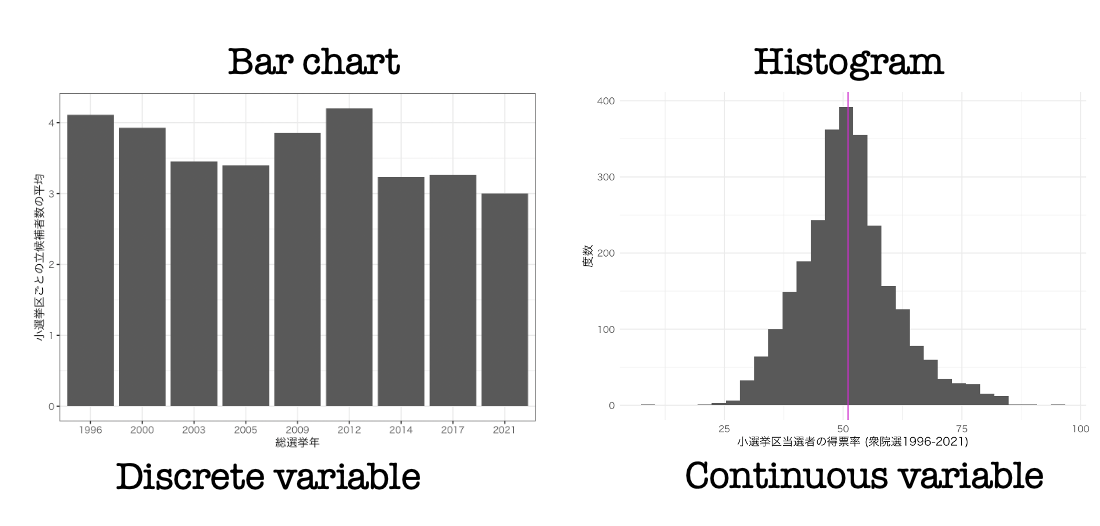• `R packages` used in this section
``````library(DT)
library(gapminder)
library(gghighlight)
library(ggrepel)
library(stargazer)
library(tidyverse)``````

# 1. Histogram

• A histogram is an approximate representation of the distribution of numerical data.

#### Diffenece between a barchart and a histogramVariable types How to visualize Feature
Discrete variable Bar chart Lines between bars
Continuous variable Histogram No Lines between bars
##### When `x-axis` is year of elections
• There is no values between 2017 and 2021 (because there was no election held between them)
• Years of election look like numeric, but it is not treated as `numeric`
##### When `x-axis` is vote share
• The value of vote share ranges from 0%, 0.1%, …. to 100%
→ We need infinite number of bars for each value
→ We do not use bars for each value
• We use limited number of bars

# 2. How to draw a histogram using ggplot2

• You can draw a histogram by using `geom_histogram()`
• You need to map a continuous variable on `x-axis`
• You don’t have to map on `y-axis`
• Let’s draw a histogram of vote share in the lower house elections in Japan between 1996 and 2021

## 2.1 Draw a simple histogram

• Make a folder, named `data` in your R Project folder
• Download hr96-21.csv onto the `data` folder in your R Project
• Read the election data, `hr96-21.csv` and name it `df`
``````df <- read_csv("data/hr96-21.csv",
na = ".")  ``````
• Using `if_else()`, make a dummy variable: `ldp`
##### `mutate(ldp = if_else(seito == "自民", "LDP", "Non-LDP")` - This command means make a dummy variable, named `ldp`
• If a value in variable `seito` is “自民”, then replace it with “LDP” and replace the other values (that is, the other party names) in `seito` with “Non-LDP”
``````df <- df %>%
mutate(ldp = if_else(seito == "自民", "LDP", "Non-LDP"))``````
• Draw a histogram of vote share
``````df %>%
ggplot() +